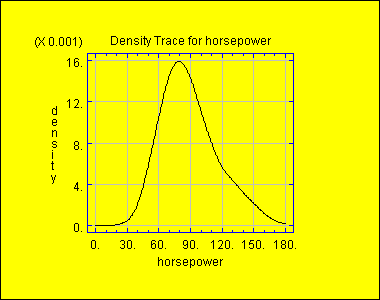# DensityTrace STATBEAN®

Purpose: This probability density function calculator estimates the probability density function for a single column of numeric data. Allows Statgraphics software to create density trace plots.

DataSource for the Probability Density Function Calculator: Any.Density Trace Read/Write Properties
 Name Type Description Possible Values Default Value columnNames String array The names of the columns (if more than one) to be analyzed. One or more strings. {""} intervalWidth int Percentage of data used to estimate trace at each location. 1-99 40 method String Type of method used to estimate the trace. "Boxcar","Cosine" "Cosine" resolution int For continuous distributions, the number of points at which the function is calculated. 50+ 300 tablewiseExclusion boolean Whether all rows of the data table containing a missing value in any column should be excluded from the plot. true,false false xVariableName String The name of the column with data values (if only one) to be plotted on the horizontal axis. Any string. ""
Probability Density Function Calculator Code Sample

//create a datasource bean
FileDataSource fileDataSource1 = new STATBEANS.FileDataSource();

//set the file name to be read
fileDataSource1.setFileName("c:\\statbeans\\samples\\cardata.txt");

//create a chart bean
DensityTrace densitytrace1 = new STATBEANS.DensityTrace();

//set the column to be plotted
densitytrace1.setXVariableName("horsepower");

//show the plot